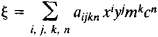# Turner's Method

## Turner’s Method

a technique used in astrophotography to determine the positions of bodies on the celestial sphere. The positions of stars, planets, artificial earth satellites, and other celestial bodies are determined on negative plates relative to reference stars, that is, stars whose equatorial coordinates are known from catalogs. Turner’s method establishes a mathematical relationship between the idealized rectangular (standard) coordinates of the reference stars, which are computed from the known equatorial coordinates of the stars, and the quasi-rectangular coordinates measured on the plates. The method was proposed by H. H. Turner in 1893.

In Turner’s method the relationship between the idealized coordinates ξ, η and the measured coordinates x, y of celestial bodies is given by the following reduction equations involving power series:

ξ = ax + by + c + dx2 + exy + fy2 + …

η = a′x + b′y + c′ + d′x2 + e′xy + f′y2 + …

where a, b,c, ..., a′, b′, c′, . . . are the reduction coefficients, which are called plate constants and are calculated by the method of least squares from systems of reduction equations constructed for the reference stars separately for ξ and η. The relations thus obtained are used to transform the coordinates x and y of a given body, which are measured on a plate, into the idealized coordinates ξ and η The latter coordinates are then used to compute the equatorial coordinates of the body.

More complicated types of reduction equations are used for modern wide-angle astrographs, for example,where aijkn are the plate constants, m is the stellar magnitude, and c is a characteristic of the spectral class of the star (a similar relation holds for η). The type of reduction equation used to determine the coordinates of a celestial body depends on the quality of the astrograph field and on the specific problem considered. Thus, if a given star and the reference stars are found within a small section of the plate, only the first three (linear) terms of the equations are used.

### REFERENCE

Podobed, V. V., and V. V. Nesterov. Obshchaia astrometriia. Moscow, 1975.
V. V. PODOBED
References in periodicals archive ?
The restoration efforts prove that Turner's method works, revealing a macaw and a shot of three children with a goldfish in vivid colour.
Turner's method requires the frequent presentation of the very language of his sources because he claims not to be searching for some "elusive social reality of extra-marital sex" (p.
It means visitors can see the various techniques used to capture views of the landscapes of the time, both in Britain and abroad, and assess Constable's and Turner's methods.
Site: Follow: Share:
Open / Close